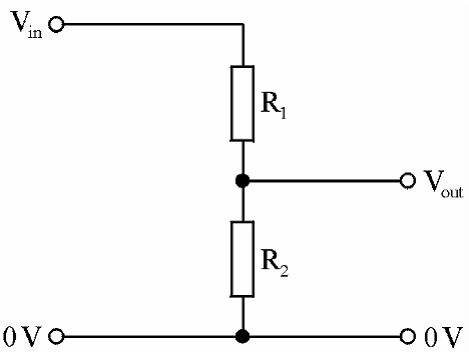Objectives:

• To identify potential divider circuits with components
• Select and use potential divider equations;$V_{out} = \frac{R_{2}}{(R_{1} + R_{2})} \times V_{in}$  and$\frac{V_{1}}{V_{2}} = \frac{R_{1}}{R_{2}}$

Potential dividers are resistors connected in series across a voltage source used to obtain a desired fraction of the voltage. An example is shown;Potential dividers are used to split the potential that is distributed to a circuit, via a cell for instance, so as to achieve a desired p.d. output. This is particularly useful if multiple outputs are required from a single e.m.f. source such as a cell.

Take a car battery as an example, the single e.m.f. source has to supply a p.d. to a number of different component such as the starter motor, headlights, radio, electric windows etc. Each of these components will likely require a different p.d. to function efficiently. Therefore a potential divider would be used supply the different p.d.’s to each device.Potential dividers are often drawn using the following diagram;The diagram is very similar to the one above however there are two terminals with$0 \ V$  and$V_{in}$  next to them. This is just a way of showing that the input voltage is supplied across this section and has a voltage value of$V_{in}$ .

Similarly the two terminals with$0 \ V$ and$V_{out}$  next to them show where the ouput p.d. would go. Ultimately you could build a new circuit at this output point and the voltage supplied to that circuit would depends on the circuit shown.

What would the output voltage be?

An equation for the output voltage can be derived using Kirchhoff’s two laws.

KCL: The sum of the current entering a junction must equal the sum of the currents leaving that same junction,$\Sigma I_{in} = \Sigma I_{out}$

KVL:  The sum of the e.m.f.’s around a loop of a circuit must equal the sum of the p.d.’s around that same loop.

Using this circuit we will derive the potential divider equation;Imagine the$V_{out}$  is simply a voltmeter measuring the p.d. across$R_{2}$

KVL: This tells us that the e.m.f. supplied$V_{IN}$  is distributed between the two resistors,$R_{1} \rightarrow V_{1}$  and$R_{2} \rightarrow V_{2}$ , so we can write;$V_{IN} = V_{1} + V_{2}$     (1)

KCL:  This tells us that the current is the same through both resistors because it is a series circuit;$I_{1} = I_{2} = I_{3}$     (2)

Since$V = IR \ \ \rightarrow \ \ I = \frac{\epsilon}{R_{T}}$ ;$I_{1} = \frac{\epsilon}{R_{T}}$$I_{2} = \frac{V_{1}}{R_{1}}$$I_{3} = \frac{V_{2}}{R_{2}}$

Subbing these three equations into equation (2);$\frac{\epsilon}{R_{T}} = \frac{V_{1}}{R_{1}} = \frac{V_{2}}{R_{2}}$     (3)

Focusing on;$\frac{\epsilon}{R_{T}} = \frac{V_{2}}{R_{2}}$

It can be rearranged for$V_{2}$ ;$\frac{\epsilon}{R_{T}}R_{2} = V_{2}$

Since$R_{T} = R_{1} + R_{2}$ ;$\frac{\epsilon}{(R_{1} + R_{2})}R_{2} = V_{2}$

This can also be written as;$V_{2} = \frac{R_{2}}{(R_{1} + R_{2})}\epsilon$

Since$\epsilon = V_{IN}$  we can write this as;$V_{2} = \frac{R_{2}}{(R_{1} + R_{2})}V_{IN}$     (4)

– – – – – – – – – – – –

Going back to equation (3) an following the same process a similar equation for$V_{1}$ can be derived;$V_{1} = \frac{R_{1}}{(R_{1} + R_{2})}V_{IN}$      (5)

– – – – – – – – – – – –

This is the equation that determines the output p.d. based on the total resistance and input voltage.

If there are more than three of more resistors this equation can be expanded further to;$V_{1} = \frac{R_{1}}{(R_{1} + R_{2} + R_{3} + ... )}V_{IN}$

– – – – – – – – – – – –

A ratio of voltages to resistances equation can be derived using equations (4) and (5) as follows, by diving equation (5) by equation (4);$\frac{V_{1}}{V_{2}} = \frac{\frac{R_{1}}{(R_{1} + R_{2})}V_{IN}}{\frac{R_{2}}{(R_{1} + R_{2})}V_{IN}}$

Although this now looks really nasty, a lot of the quantities cancels out and then leaves;$\frac{V_{1}}{V_{2}} = \frac{R_{1}}{R_{2}}$